### Home > CALC > Chapter 4 > Lesson 4.2.4 > Problem4-93

4-93.
1. In Chapter 4, it was discovered that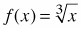was not differentiable at x = 0. Homework Help ✎

1. Why does the derivative ofnot exist at x = 0?

2. Is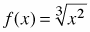differentiable at x = 0? Why or why not?

3. What about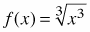?

4. Explain why there is a point of inflection at x = 0 for.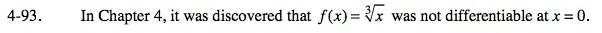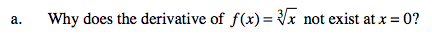What is the slope of the function at this point? Can you compute it?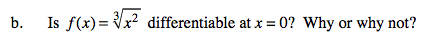Graph the function or evaluate the derivative at 0.

No, the tangent is still vertical (and in this case there is a cusp).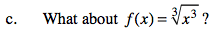Simplify f(x).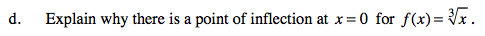What happens on either side of a point of inflection?What kind of change does a point of inflection signify?

Even though f ''(0) = DNE, there is still a point of inflection because the graph changes from concave up to concave down at x = 0.